SPSS TUTORIALS BASICS ANOVA REGRESSION FACTOR CORRELATION

# SPSS Stepwise Regression – Simple Tutorial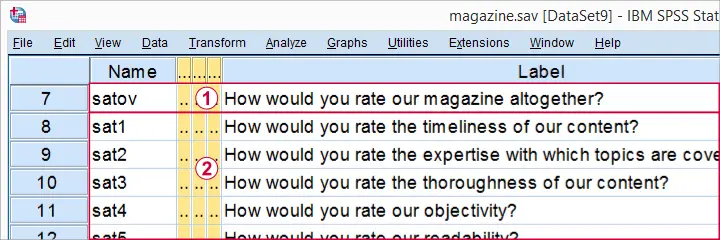A magazine wants to improve their customer satisfaction. They surveyed some readers ontheir overall satisfaction as well assatisfaction with some quality aspects. Their basic question is “which aspects have most impact on customer satisfaction?” We'll try to answer this question with regression analysis. Overall satisfaction is our dependent variable (or criterion) and the quality aspects are our independent variables (or predictors).
These data -downloadable from magazine_reg.sav- have already been inspected and prepared in Stepwise Regression in SPSS - Data Preparation.

## Preliminary Settings

Our data contain a FILTER variable which we'll switch on with the syntax below. We also want to see both variable names and labels in our output so we'll set that as well.

*1. Switch filter variable on.

filter by filt1.

*2. Show variable names and labels in output.

set tvars both.

## SPSS ENTER Regression

We'll first run a default linear regression on our data as shown by the screenshots below.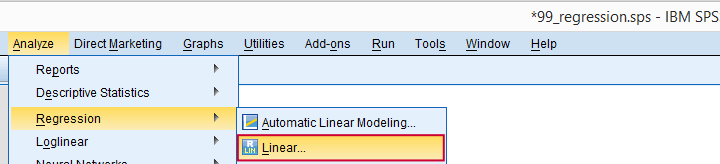Let's now fill in the dialog and subdialogs as shown below.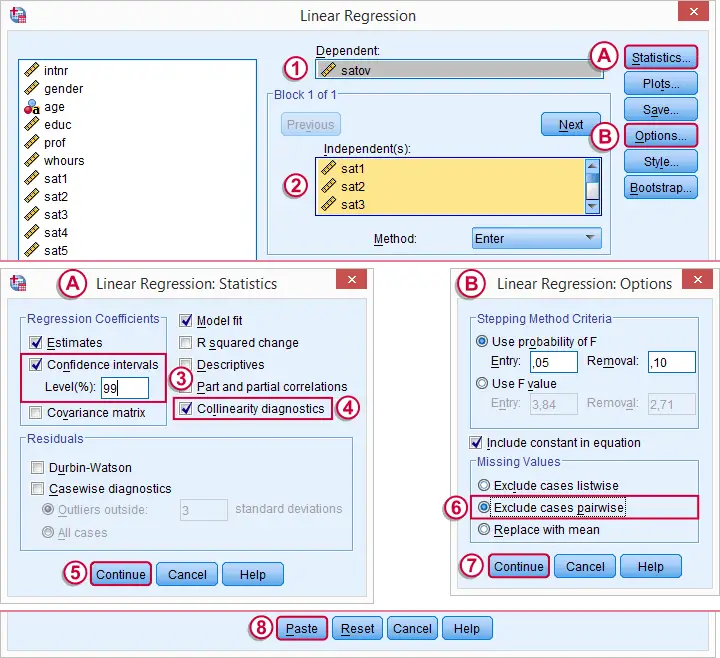Note that we usually select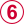Exclude cases pairwise because it uses as many cases as possible for computing the correlations on which our regression is based.

Clicking results in the syntax below. We'll run it right away.

## SPSS ENTER Regression - Syntax

*Basic regression syntax. Note that "method=enter" in last line.

REGRESSION
/MISSING PAIRWISE
/STATISTICS COEFF CI(99) OUTS R ANOVA COLLIN TOL
/CRITERIA=PIN(.05) POUT(.10)
/NOORIGIN
/DEPENDENT satov
/METHOD=ENTER sat1 sat2 sat3 sat4 sat5 sat6 sat7 sat8 sat9.

## SPSS ENTER Regression - Output

In our output, we first inspect our coefficients table as shown below.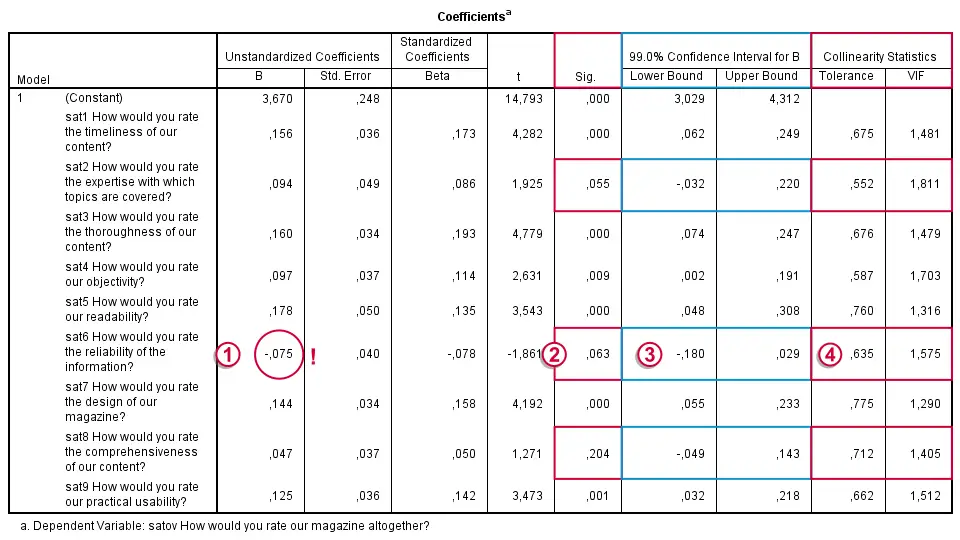Some things are going dreadfully wrong here:The b-coefficient of -0.075 suggests that lower “reliability of information” is associated with higher satisfaction. However, these variables have a positive correlation (r = 0.28 with a p-value of 0.000).This weird b-coefficient is not statistically significant: there's a 0.063 probability of finding this coefficient in our sample if it's zero in the population. This goes for some other predictors as well.

This problem is known as multicollinearity: we entered too many intercorrelated predictors into our regression model. The (limited) r square gets smeared out over 9 predictors here. Therefore, the unique contributions of some predictors become so small that they can no longer be distinguished from zero.The confidence intervals confirm this: it includes zero for three b-coefficients.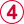A rule of thumb is that Tolerance < 0.10 indicates multicollinearity. In our case, the Tolerance statistic fails dramatically in detecting multicollinearity which is clearly present. Our experience is that this is usually the case.

## Resolving Multicollinearity with Stepwise Regression

A method that almost always resolves multicollinearity is stepwise regression. We specify which predictors we'd like to include. SPSS then inspects which of these predictors really contribute to predicting our dependent variable and excludes those who don't.
Like so, we usually end up with fewer predictors than we specify. However, those that remain tend to have solid, significant b-coefficients in the expected direction: higher scores on quality aspects are associated with higher scores on satisfaction. So let's do it.

## SPSS Stepwise Regression - Syntax

We copy-paste our previous syntax and set `METHOD=STEPWISE` in the last line. Like so, we end up with the syntax below. We'll run it and explain the main results.

*Basic stepwise regression.

REGRESSION
/MISSING PAIRWISE
/STATISTICS COEFF OUTS CI(99) R ANOVA
/CRITERIA=PIN(.05) POUT(.10)
/NOORIGIN
/DEPENDENT satov
/METHOD=stepwise sat1 sat2 sat3 sat4 sat5 sat6 sat7 sat8 sat9.

## SPSS Stepwise Regression - Variables Entered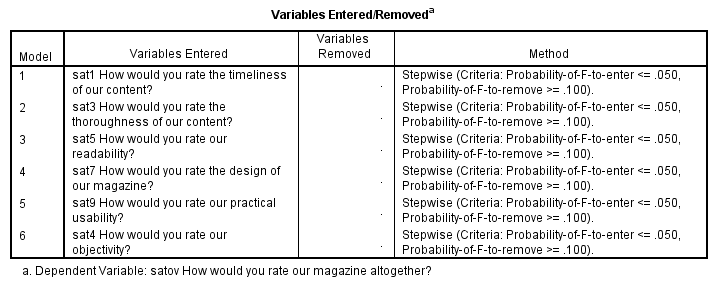This table illustrates the stepwise method: SPSS starts with zero predictors and then adds the strongest predictor, sat1, to the model if its b-coefficient in statistically significant (p < 0.05, see last column).
It then adds the second strongest predictor (sat3). Because doing so may render previously entered predictors not significant, SPSS may remove some of them -which doesn't happen in this example.
This process continues until none of the excluded predictors contributes significantly to the included predictors. In our example, 6 out of 9 predictors are entered and none of those are removed.

## SPSS Stepwise Regression - Model Summary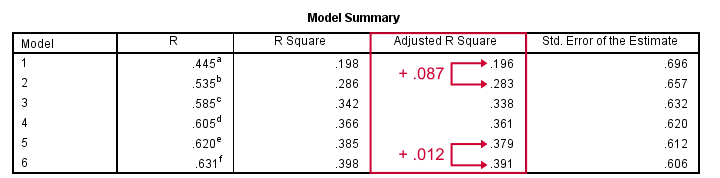SPSS built a model in 6 steps, each of which adds a predictor to the equation. While more predictors are added, adjusted r-square levels off: adding a second predictor to the first raises it with 0.087, but adding a sixth predictor to the previous 5 only results in a 0.012 point increase. There's no point in adding more than 6 predictors.
Our final adjusted r-square is 0.39, which means that our 6 predictors account for 39% of the variance in overall satisfaction. This is somewhat disappointing but pretty normal in social science research.

## SPSS Stepwise Regression - Coefficients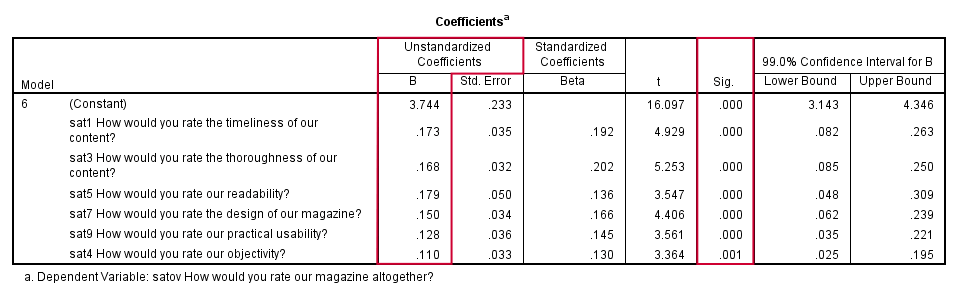In our coefficients table, we only look at our sixth and final model. Like we predicted, our b-coefficients are all significant and in logical directions. Because all predictors have identical (Likert) scales, we prefer interpreting the b-coefficients rather than the beta coefficients. Our final model states that satov’ = 3.744 + 0.173 sat1 + 0.168 sat3 + 0.179 sat5
+ 0.150 sat7 + 0.128 sat9 + 0.110 sat4
Our strongest predictor is sat5 (readability): a 1 point increase is associated with a 0.179 point increase in satov (overall satisfaction). Our model doesn't prove that this relation is causal but it seems reasonable that improving readability will cause slightly higher overall satisfaction with our magazine.

# Tell us what you think!

*Required field. Your comment will show up after approval from a moderator.

# THIS TUTORIAL HAS 15 COMMENTS:

•### By Ruben Geert van den Berg on March 22nd, 2018

Hi Lisa!

With real world data, you can't draw that conclusion.

The problem is that predictors are usually correlated. So some of the variance explained by predictor A is also explained by predictor B. To which predictor are you going to attribute that?

That is, if A has r-square = 0.3 and B has r-square = 0.3, then A and B usually have r-square lower than 0.6 because they overlap.

Most of the variance explained by the entire regression equation can be attributed to several predictors simultaneously. So the truly unique contributions to r-square don't add up to the total r-square unless all predictors are uncorrelated -which never happens.

A better idea is to add up the beta coefficients and see what percentage of this sum each predictor constitutes. Or do the same thing with B coefficients if all predictors have identical scales (such as 5-point Likert).

Last, keep in mind that regression does not prove any causal relations.

So b = 1 means that one unit increase in b is associated with one unit increase in y (correlational statement).

You can not conclude that one unit increase in b will result in one unit increase in y (causal statement). In fact, the latter will rarely be the case.

Hope that helps!

•### By Lisa on March 23rd, 2018

Thank you! It is much clearer now. Just one more quick question please :) What is the correct way to interpret the data where the b coefficient is x% of total coefficients?

•### By Ruben Geert van den Berg on March 23rd, 2018

I'd simply say something like "factor A accounts for ...% of the total impact on ...".

Which is technically not entirely correct. But it may be the best answer you can give to the question being asked.

Especially in market research, your client may be happier with an approximate answer than a complicated technical explanation -perhaps 100% correct- that does not answer the question at all because it strictly can't be answered. In such cases, being a little less strict probably gets you further.

•### By Colin Atkinson on November 8th, 2022

An excellent introduction to logistic regression. Thank you.

•### By Isyaku Ibrahim on December 28th, 2022

Excellent tutorial please keep it up